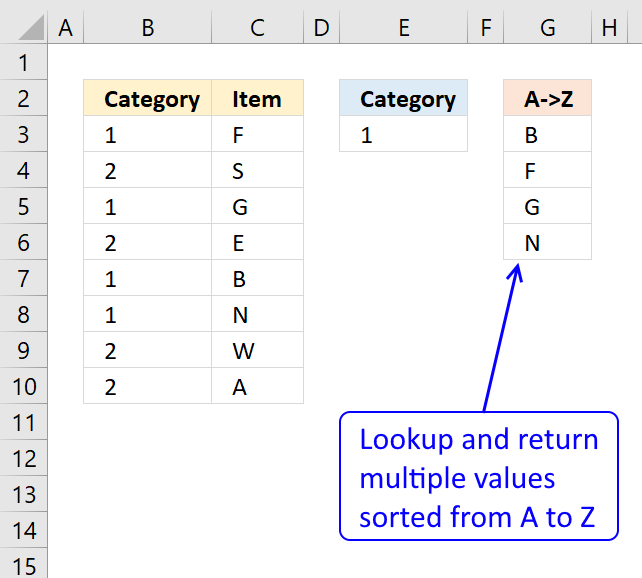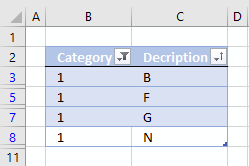Author: Oscar Cronquist Article last updated on February 14, 2018

The array formula in column G filters values in column C using a condition in cell E3, comparing it with values in adjacent column B. The filtered values are then sorted from A to Z.It is possible to build a formula around the VLOOKUP function but it would be big, the following formula is smaller and easier to understand.

Array formula in cell G3:

=INDEX(\$C\$3:\$C\$10, MATCH(SMALL(IF(\$E\$3=\$B\$3:\$B\$10, COUNTIF(\$C\$3:\$C\$10, "<"&\$C\$3:\$C\$10), ""),ROWS(\$A\$1:A1)), COUNTIF(\$C\$3:\$C\$10,"<"&\$C\$3:\$C\$10), 0))

Watch a video where I explain the formula

Recommended article

Unique distinct list sorted alphabetically based on a condition

The array formula in cell E6 filters values in column C based on value in cell E3, the output is […]

How to enter an array formula

1. Double click on cell G3
2. Copy and paste above formula to cell G3
3. Press and hold CTRL + SHIFT simultaneously
4. Press Enter once
1. Release all keys

Examine the formula bar and you will see that the formula now has a beginning and ending curly bracket. Don't enter these characters yourself, they appear automatically. Example, {=array_formula}Recommended article

How to enter an array formula

Array formulas allows you to do advanced calculations not possible with regular formulas.

Explaining formula in cell G3

Step 1 - Sort values in column C

COUNTIF(\$C\$3:\$C\$10, "<"&\$C\$3:\$C\$10)

becomes

COUNTIF({"F"; "S"; "G"; "E"; "B"; "N"; "W"; "A"},{"<F"; "<S"; "<G"; "<E"; "<B"; "<N"; "<W"; "<A"})

and returns

{3;6;4;2;1;5;7;0}

Recommended article

How to use the COUNTIF function

Counts the number of cells that meet a specific condition.

Step 2 - Extract sort rank numbers for chosen category

IF(\$E\$3=\$B\$3:\$B\$10, COUNTIF(\$C\$3:\$C\$10, "<"&\$C\$3:\$C\$10), "")

becomes

IF(\$E\$3=\$B\$3:\$B\$10, {3;6;4;2;1;5;7;0}, "")

becomes

IF(\$E\$3=\$B\$3:\$B\$10, {3;6;4;2;1;5;7;0}, "")

becomes

IF(1={1;2;1;2;1;1;2;2},{3;6;4;2;1;5;7;0},"")

becomes

IF({TRUE;FALSE;TRUE;FALSE;TRUE;TRUE;FALSE;FALSE},{3;6;4;2;1;5;7;0},"")

and returns

{3;"";4;"";1;5;"";""}

Recommended article

How to use the IF function

Checks if a logical expression is met. Returns a specific value if TRUE and another specific value if FALSE.

Step 3 - Find k-th smallest value in array

SMALL(IF(\$E\$3=\$B\$3:\$B\$10,COUNTIF(\$C\$3:\$C\$10,"<"&\$C\$3:\$C\$10),""),ROWS(\$A\$1:A1))

becomes

SMALL({3;"";4;"";1;5;"";""},ROWS(\$A\$1:A1))

becomes

SMALL({3;"";4;"";1;5;"";""},1)

and returns 1.

Recommended article

How to use the SMALL function

The SMALL function lets you extract a number in a cell range based on how small it is compared to the other numbers in the group.

Step 4 - Match sort rank to find relative position

MATCH(SMALL(IF(\$E\$3=\$B\$3:\$B\$10, COUNTIF(\$C\$3:\$C\$10, "<"&\$C\$3:\$C\$10), ""),ROWS(\$A\$1:A1)), COUNTIF(\$C\$3:\$C\$10,"<"&\$C\$3:\$C\$10), 0)

becomes

MATCH(1, {3;6;4;2;1;5;7;0}, 0)

and returns 5.

Recommended article

How to use the MATCH function

Identify the position of a value in an array.

Step 5 - Return values

INDEX(\$C\$3:\$C\$10,MATCH(SMALL(IF(\$E\$3=\$B\$3:\$B\$10,COUNTIF(\$C\$3:\$C\$10,"<"&\$C\$3:\$C\$10),""),ROWS(\$A\$1:A1)),COUNTIF(\$C\$3:\$C\$10,"<"&\$C\$3:\$C\$10),0))

becomes

INDEX(\$C\$3:\$C\$10,5)

becomes

INDEX({"F";"S";"G";"E";"B";"N";"W";"A"},5)

and returns B in cell G3.

Recommended article

How to use the INDEX function

Gets a value in a specific cell range based on a row and column number.

Tip! You can easily filter values if you convert your data to an excel table and then sort them:Become more productive – Learn Excel Defined Tables

An Excel table allows you to easily sort, filter and sum values in a data set where values are related.

Use VLOOKUP and return multiple values sorted from A to Z.xlsx

Recommended articles

Sort a column alphabetically

Table of Contents Sort a column using array formula Two columns sorting by the second column Sort alphanumeric values I […]

5 easy ways to VLOOKUP and return multiple values

This post explains how to lookup a value and return multiple values. No array formula required.

Unique distinct records sorted by frequency

Sara asks: How can you use large with multiple criteria?? Example looking for top 5 of a list based on […]

Sort column based on frequency

Question: How do I create a new unique distinct list from a column. I also want the list sorted from large […]

Create a drop down list containing alphabetically sorted values

This article describes how to create a drop-down list populated with sorted values from A to Z. The sorted list […]

Create a unique distinct sorted list containing both numbers text removing blanks

The image above demonstrates a formula in cell D3 that extracts unique distinct numbers and text values sorted from A […]